CAT  >  IIFT Logical Reasoning MCQ Quiz - 2

# IIFT Logical Reasoning MCQ Quiz - 2

Test Description

## 20 Questions MCQ Test IIFT Mock Test Series | IIFT Logical Reasoning MCQ Quiz - 2

IIFT Logical Reasoning MCQ Quiz - 2 for CAT 2022 is part of IIFT Mock Test Series preparation. The IIFT Logical Reasoning MCQ Quiz - 2 questions and answers have been prepared according to the CAT exam syllabus.The IIFT Logical Reasoning MCQ Quiz - 2 MCQs are made for CAT 2022 Exam. Find important definitions, questions, notes, meanings, examples, exercises, MCQs and online tests for IIFT Logical Reasoning MCQ Quiz - 2 below.
Solutions of IIFT Logical Reasoning MCQ Quiz - 2 questions in English are available as part of our IIFT Mock Test Series for CAT & IIFT Logical Reasoning MCQ Quiz - 2 solutions in Hindi for IIFT Mock Test Series course. Download more important topics, notes, lectures and mock test series for CAT Exam by signing up for free. Attempt IIFT Logical Reasoning MCQ Quiz - 2 | 20 questions in 20 minutes | Mock test for CAT preparation | Free important questions MCQ to study IIFT Mock Test Series for CAT Exam | Download free PDF with solutions
 1 Crore+ students have signed up on EduRev. Have you?
IIFT Logical Reasoning MCQ Quiz - 2 - Question 1

### Ashika is taller than Ashna. Ashna is lighter than Ashuka. Ashuka is heavier than Arushi. Arushi is taller than Ashika. Arushi is heavier than Ashika. Based on the above statements, which of the following is definitely true?

Detailed Solution for IIFT Logical Reasoning MCQ Quiz - 2 - Question 1

From the given statements, it can be inferred that: In terms of height: Arushi > Ashika > Ashna In terms of weight: Ashuka > Arushi > Ashika and Ashuka > Ashna Therefore, statements of options 2 and 3 are false.

Option 1 may or may not be true.

Hence, option 4.

IIFT Logical Reasoning MCQ Quiz - 2 - Question 2

### Complete the following series: A, D, 1, B, G, 3, C, L, 7, D , __, __

Detailed Solution for IIFT Logical Reasoning MCQ Quiz - 2 - Question 2

Considering every third term, the given series can be split into 3 parts: (A, B, C, D), (D, G, L , __) and (1, 3, 7 , __)

Consider the difference between consecutive terms in the third part: 3 - 1 = 2 and 7 - 3 = 4

Since the difference is 2, 4 , t he next difference can be 6 (if the differences form an A.P) or 8 (if the differences form a G.P.).

For a difference of 6, the next number should be 7 + 6 = 13 and a for a difference of 8, the next number should be 7 + 8 = 15.

However, none of the options has 13 as the required number. So, the missing number is 15.
Hence, options 1 and 4 can be eliminated.

Consider D, G, L , __.
Their position in the alphabet is 4, 7, 12...and the difference between them is 3, 5,...

So, the next difference should be 7.
So, the missing letter should be the 12 + 7 i.e. 19th letter of the alphabet. Thus, the missing letter should be S.

Hence, option 3.

IIFT Logical Reasoning MCQ Quiz - 2 - Question 3

### Four brothers - Udyam, Udyan, Antony and Uttam bought a piece of diamond weighing 720 g for Rs. 2,40,000. Udyam paid half of the amount paid by the other three brothers. Udyan paid one-fifth of the amount paid by the other three brothers. The amount paid by Antony was five-nineteenth of the amount paid by the other three brothers. If each of them claimed their stake in the diamond in proportion to the amount they paid, then what is the weight of the diamond piece that Uttam will get?

Detailed Solution for IIFT Logical Reasoning MCQ Quiz - 2 - Question 3

Let the amount paid by Udyam, Udyan, Antony and Uttam be Rs. a, b, c, and d respectively.

Let the price of the diamond be Rs. k.

We know that a: (b + c + d)= 1 :2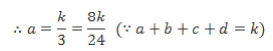We know that b :(a + c + d) = 1 : 5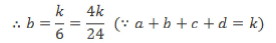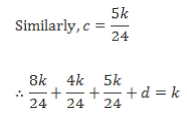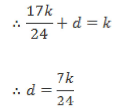∴ The amounts paid by Udyam, Udyan, Antony and Uttam are in the ratio 8 : 4 : 5 : 7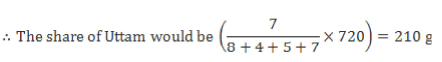Hence, option 1.

IIFT Logical Reasoning MCQ Quiz - 2 - Question 4

The oldest daughter of Melissa is Sita. Sita has one brother, Rama, and one sister, Savia. Melissa’s only sister, Rajalakshmi, has two children: son Kannan, and daughter, Radha. If Geetha is Savia’s only daughter, then Sita’s husband is Geetha’s :

Detailed Solution for IIFT Logical Reasoning MCQ Quiz - 2 - Question 4

The given data can be represented as shown below: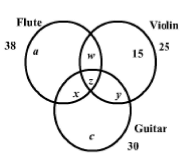a + 15 + c = 55 ...(i)

w + y + z + 15 = 25

∴ w + y + z = 10 ...(ii)

a + x + w + z = 38 ...(iii)

∴ c = 30 - (6 + 3 + 6) = 15

∴ a = 55 - ( 1 5 + 15) = 25 Thus, the entire Venn diagram can be filled as shown below: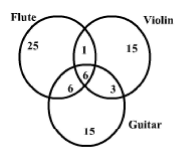∴ Total number of students = 25+ 15+ 15+ 1+ 6 + 3 + 6 = 71 Hence, option 2.

IIFT Logical Reasoning MCQ Quiz - 2 - Question 5

Answer the following question based on the information given below.

A word arrangement machine, when given a particular input, rearranges it using a particular rule. The following two examples are the illustration and the steps of the arrangement Input:

KAT CAT JAT VAT

STEP 1: JAT KAT CAT VAT

STEP 2: VAT CAT KAT JAT

STEP 3: KAT JAT VAT CAT

STEP 4: CAT VAT JAT KAT

Input: MAT JAT BAT UAT

STEP 1: JAT BAT UAT MAT

STEP 2: MAT UAT BAT JAT

STEP 3: BAT JAT MAT UAT

STEP 4: UAT MAT JAT BAT

Q.

Which of the following will be step 1 for the following input?

MAT WAT OAT GAT

Detailed Solution for IIFT Logical Reasoning MCQ Quiz - 2 - Question 5

We can easily recognize that each word given to the machine has three letters and the last two letters of each word are same.

Hence, words are arranged based on the relation between the first letters of those words.

After proper evaluation of a problem, we can see the following pattern: If we assign a number to each letter as they appear in alphabetical order, For example A =1, B = 2, C = 3 and so on.

J is the 10th letter; therefore for J we will consider value 1 + 0 = 1 K is the 11th letter; therefore for K we will consider value 1 + 1 = 2 and so on.
Hence, we can assign the following values to each letter.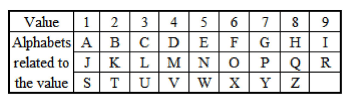We can see that in the first step, the words are arranged in the increasing order of their values.

In the second step, position of the 1st word of step 1 is interchanged with that of the 4th word whereas the position of the 2nd word is interchanged with that of the 3rd word.

In the third step, position of 1st and 3rd words; and 2nd and 4th words of step 2 are interchanged.
In the fourth step, position of 1st and 4th words; and 2nd and 3rd words of step 3 are interchanged.
Hence for the given input:

STEP 1: MAT WAT OAT GAT

Hence, option 1.

IIFT Logical Reasoning MCQ Quiz - 2 - Question 6

Answer the following question based on the information given below.

A word arrangement machine, when given a particular input, rearranges it using a particular rule. The following two examples are the illustration and the steps of the arrangement Input:

KAT CAT JAT VAT

STEP 1: JAT KAT CAT VAT

STEP 2: VAT CAT KAT JAT

STEP 3: KAT JAT VAT CAT

STEP 4: CAT VAT JAT KAT

Input: MAT JAT BAT UAT

STEP 1: JAT BAT UAT MAT

STEP 2: MAT UAT BAT JAT

STEP 3: BAT JAT MAT UAT

STEP 4: UAT MAT JAT BAT

Q.

Which of the following will be step 4 for the following input?

FAT ZAT RAT SAT

Detailed Solution for IIFT Logical Reasoning MCQ Quiz - 2 - Question 6

Going by the logic mentioned in the solution to the fisrt question of this set,

Step 1: SAT FAT ZAT RAT

Step 2: RAT ZAT FAT SAT

Step 3: FAT SAT RAT ZAT

Step 4: ZAT RAT SAT FAT

Hence, option 3.

IIFT Logical Reasoning MCQ Quiz - 2 - Question 7

Answer the following question based on the information given below.

A word arrangement machine, when given a particular input, rearranges it using a particular rule. The following two examples are the illustration and the steps of the arrangement Input:

KAT CAT JAT VAT

STEP 1: JAT KAT CAT VAT

STEP 2: VAT CAT KAT JAT

STEP 3: KAT JAT VAT CAT

STEP 4: CAT VAT JAT KAT

Input: MAT JAT BAT UAT

STEP 1: JAT BAT UAT MAT

STEP 2: MAT UAT BAT JAT

STEP 3: BAT JAT MAT UAT

STEP 4: UAT MAT JAT BAT

Q.

Which of the following will be step 2 for the following input?

OAT HAT EAT TAT

Detailed Solution for IIFT Logical Reasoning MCQ Quiz - 2 - Question 7

Going by the logic mentioned in the solution to the first question of this set,

Step 1: TAT EAT OAT HAT

Step 2: HAT OAT EAT TAT

Hence, option 4.

IIFT Logical Reasoning MCQ Quiz - 2 - Question 8

Answer the following question based on the information given below.

A word arrangement machine, when given a particular input, rearranges it using a particular rule. The following two examples are the illustration and the steps of the arrangement Input:

KAT CAT JAT VAT

STEP 1: JAT KAT CAT VAT

STEP 2: VAT CAT KAT JAT

STEP 3: KAT JAT VAT CAT

STEP 4: CAT VAT JAT KAT

Input: MAT JAT BAT UAT

STEP 1: JAT BAT UAT MAT

STEP 2: MAT UAT BAT JAT

STEP 3: BAT JAT MAT UAT

STEP 4: UAT MAT JAT BAT

Q.

There are four arguments I, II, III and IV, each comprising three statements. In how many of the given arguments does the third statement logically Marks follow from the first two statements.

I. Some A are B. All B are C. Some A are C.
II. All temples are red. No red is blue. No temple is blue.
III. No apple is an orange. Some oranges are rotten. Some apples are rotten.
IV. Every bicycle has wheels. All wheels are round. No bicycle is round.

Detailed Solution for IIFT Logical Reasoning MCQ Quiz - 2 - Question 8

Consider Argument IV: Since every bicycle has wheels and all wheels are round, all bicycles are round.

So, the third statement in argument IV does not follow from the first two. Consider Argument I: Some A are B and all B are C.

So, all the A that are B have to be C as well. So, there are definitely some A that are C.

So, the third statement in argument I follows from the first two.

Consider Argument II: All temples are red and no red is blue.
So, no temple can be blue (as it is red).

So, the third statement in argument II follows from the first two.

Consider Argument III: No apple is an orange but some oranges are rotten.

So, there exists a case where the set of oranges and the set of rotten things intersect partially. In such a case, the set of apples can also intersect with the set of rotten things (without intersecting the set of oranges).

So, the third statement may or may not be true. Hence, it cannot be said to follow from the first two.
Thus, the third statement follows from the first two for two arguments (I and II).

Hence, option 3.

IIFT Logical Reasoning MCQ Quiz - 2 - Question 9

A committee of 5 students has to be made for the management fest. A, B, C, D, E, F, G, H, I, and J are 10 students nominated for the 5 positions. Only 1 out of A, B and C can and should be selected. If D is selected, E is not selected. If both E and F are selected then H is not selected. Find the number of different committees that can be formed if it is known that only one student out of I and J must be selected.

Detailed Solution for IIFT Logical Reasoning MCQ Quiz - 2 - Question 9

One student out of A, B, C can be selected in 3C1 ways = 3 ways One student out of I and J can be selected in 2C1 ways = 2 ways Now, there are 2 possible cases: Case 1: E is selected.
∴ D is not selected.

If E and F are selected then H is not selected.

∴  Either (E, G, H) or (E, F, G) are selected.

∴ 2 ways of selection are possible.
Case 2: E is not selected.

∴  Three students out of D, F, G, H can be selected in 4C3 ways = 4 ways Therefore, the number of different committees that can be formed = 3 x 2 x (2 + 4 ) = 36 ways.

Hence, option 3.

IIFT Logical Reasoning MCQ Quiz - 2 - Question 10

A King Baton Relay has to be passed through 5 Countries P, Q R, S and T. It should start at P and end at T and should visit every country only once. Q is at 60 km west from P R is at 11 km south from Q. S is 22 km north from R and finally T is at 120 km west from P.

If the speed during the whole journey is constant then what will be the minimum distance travelled by relay from P to T?

Detailed Solution for IIFT Logical Reasoning MCQ Quiz - 2 - Question 10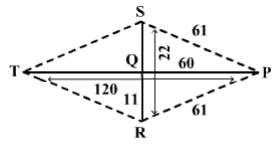From the figure, it can be seen that considering all the given conditions there are only the following two ways to travel from P to T.

(i) P - S - Q - R - T o r

(ii) P - R - Q - S - T

For (i) the total distance travelled is

= PS + SQ + QR + RT

= 61 + 11 + 11 + 61 = 144 km.

For (ii) the total distance travelled is

= PR + RQ + QS + ST

= 61 + 11 + 11 + 61 = 144 km.

As both the distances are same, the minimum distance travelled by the relay is 144 km.

Hence, option 3.

IIFT Logical Reasoning MCQ Quiz - 2 - Question 11

Shankar, Sunil, and Suman are three friends each having different professions and each being either tall or dark or handsome. Among them Suman is older than the handsome one but younger than the businessman. Shankar is younger than the dark one but Sunil is older than the tall one. The martial arts instructor is the actor’s younger brother. Which of the following statements is true?

Detailed Solution for IIFT Logical Reasoning MCQ Quiz - 2 - Question 11

It is given that Suman is older than the handsome one but younger than the businessman.

This implies that Suman is second in terms of age

∴ Handsome is the characterstic of the youngest person and businessman is the profession of the oldest person.

The Martial Arts instructor is the Actor’s younger brother and the businessman is the oldest person. .'.

∴ The actor is the second oldest.

∴ Suman is the actor and the youngest person is a martial arts instructor,

∵ It is given that Shankar is younger than the dark one.

∴ Shankar is not dark and not the oldest.
∴ He is the youngest and the martial arts instructor and hence handsome.

∵ Sunil is older than the tall one.

∴ Sunil is the oldest and the dark one.

∴ Suman is the tall one.

∴ Only option 3 is true.

Hence, option 3.

IIFT Logical Reasoning MCQ Quiz - 2 - Question 12

If AN : 13, BE : 5, RAHUL : 55, then RITESH = ?

Detailed Solution for IIFT Logical Reasoning MCQ Quiz - 2 - Question 12

The position of A, N in the alphabet is 1, 14 respectively.

Now, 1 + 14 = 15

But, AN = 13 (= 15 - 2)

The position of B, E in the alphabet is 2, 5 respectively.
Now, 2 + 5 = 7

But, BE = 5 (= 7 - 2) Marks

The position of R, A, H, U, L in the alphabet is 18, 1,8, 21, 12 respectively. Now, 18 + 1 + 8 + 21 + 12 = 60 But, RAHUL = 55

Note that the words AN, BE and RAHUL has 2, 2 and 5 letters respectively.

Therefore, the pattern followed is:

Word = Sum of position of letters - Number of letters in the word

RITESH = (18 + 9 + 20 + 5 + 19 + 8) - 6 = 73

Hence, option 1.

IIFT Logical Reasoning MCQ Quiz - 2 - Question 13

Answer the following question based on the information given below.
Six classes, namely: Class A, Class B, Class C, Class D, Class E and Class F of a certain Management Institute were supposed to select exactly three specializations from Marketing, Finance, Human Resources, IT Management, Operations Management and Retail Management. All the students in any particular class selected the same set of specializations and further each specialization was selected by atleast one of the six classes. Also, 1. Students of Class B selected exactly one specialization which the students of Class E rejected. 2. There were exactly two specializations that were chosen by Class A as well as Class C. 3. There were exactly three specializations that were not chosen by Class C as well as Class F. 4. The students of Class F chose Marketing and Operations Management while the students of Class E did not choose IT Management.
The table below gives the details of the number of classes that opted for the specializations.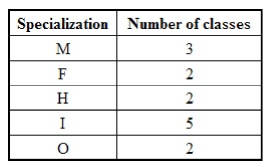In the above table: M: Marketing, F: Finance, H: Human Resources, I: IT Management, O: Operations Management.

Q.

What would be the value of H2 +R2?

Detailed Solution for IIFT Logical Reasoning MCQ Quiz - 2 - Question 13

The number of specializations chosen by each of the class is exactly three.

Hence, we have: M + F + H + I + 0 + R= 18.

Hence, R = 4.

R2 + H2 = 42 + 22 = 20
Hence, option 3.

IIFT Logical Reasoning MCQ Quiz - 2 - Question 14

Answer the following question based on the information given below.
Six classes, namely: Class A, Class B, Class C, Class D, Class E and Class F of a certain Management Institute were supposed to select exactly three specializations from Marketing, Finance, Human Resources, IT Management, Operations Management and Retail Management. All the students in any particular class selected the same set of specializations and further each specialization was selected by atleast one of the six classes. Also, 1. Students of Class B selected exactly one specialization which the students of Class E rejected. 2. There were exactly two specializations that were chosen by Class A as well as Class C. 3. There were exactly three specializations that were not chosen by Class C as well as Class F. 4. The students of Class F chose Marketing and Operations Management while the students of Class E did not choose IT Management.
The table below gives the details of the number of classes that opted for the specializations.In the above table: M: Marketing, F: Finance, H: Human Resources, I: IT Management, O: Operations Management.

Q.

In case of how many classes, can the three specializations chosen by them be uniquely determined?

Detailed Solution for IIFT Logical Reasoning MCQ Quiz - 2 - Question 14

The number of specializations chosen by each of the class is exactly three. H ence, w e have: M + F + H + I + 0 + R = 1 8 .

Hence, R = 4.

Class E did not choose IT Management and since the number of classes that chose IT Management is 5; we can conclude that all the other classes chose IT Management.
Students of Class F chose Marketing and Operations Management. There are exactly three specializations which have not been chosen by Class C as well as Class F implies that both Class C and Class F chose the same specializations, i.e. Marketing, Operations Management and IT Management.

Since, Operations Management was chosen by only 2 classes, none of the other classes chose Operations Management. Now between Class A and C, there were exactly two specializations which were same, implies that Class A chose Marketing apart from IT Management.

Also since Class C and Class F did not choose Retail implies that all the remaining classes had chosen Retail Management as one of their specializations.

The above information can be tabulated as follows: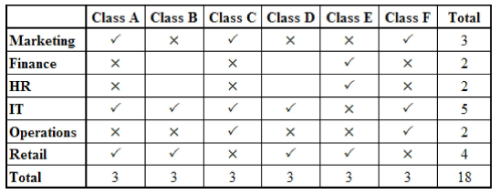∴ The number of classes for whom the specializations taken can be uniquely determined is 4.

Hence, option 4.

IIFT Logical Reasoning MCQ Quiz - 2 - Question 15

Answer the following question based on the information given below.
Six classes, namely: Class A, Class B, Class C, Class D, Class E and Class F of a certain Management Institute were supposed to select exactly three specializations from Marketing, Finance, Human Resources, IT Management, Operations Management and Retail Management. All the students in any particular class selected the same set of specializations and further each specialization was selected by atleast one of the six classes. Also, 1. Students of Class B selected exactly one specialization which the students of Class E rejected. 2. There were exactly two specializations that were chosen by Class A as well as Class C. 3. There were exactly three specializations that were not chosen by Class C as well as Class F. 4. The students of Class F chose Marketing and Operations Management while the students of Class E did not choose IT Management.
The table below gives the details of the number of classes that opted for the specializations.In the above table: M: Marketing, F: Finance, H: Human Resources, I: IT Management, O: Operations Management.

Q.

Which of the following specializations has been definitely chosen by students of Class A?

Detailed Solution for IIFT Logical Reasoning MCQ Quiz - 2 - Question 15

From the table in the solution to the first question of this set, we can see that Class A definitely chose Marketing and IT Management.

Hence, option 4.

IIFT Logical Reasoning MCQ Quiz - 2 - Question 16

Answer the following question based on the information given below.
Six classes, namely: Class A, Class B, Class C, Class D, Class E and Class F of a certain Management Institute were supposed to select exactly three specializations from Marketing, Finance, Human Resources, IT Management, Operations Management and Retail Management. All the students in any particular class selected the same set of specializations and further each specialization was selected by atleast one of the six classes. Also, 1. Students of Class B selected exactly one specialization which the students of Class E rejected. 2. There were exactly two specializations that were chosen by Class A as well as Class C. 3. There were exactly three specializations that were not chosen by Class C as well as Class F. 4. The students of Class F chose Marketing and Operations Management while the students of Class E did not choose IT Management.
The table below gives the details of the number of classes that opted for the specializations.In the above table: M: Marketing, F: Finance, H: Human Resources, I: IT Management, O: Operations Management.

Q.

Which of the following chose Operations Management?

Detailed Solution for IIFT Logical Reasoning MCQ Quiz - 2 - Question 16

From the table in the solution to the first question of this set, we can see that C and F chose Operations Management.

Hence, option 2.

IIFT Logical Reasoning MCQ Quiz - 2 - Question 17

Answer the following question based on the information given below.
Six classes, namely: Class A, Class B, Class C, Class D, Class E and Class F of a certain Management Institute were supposed to select exactly three specializations from Marketing, Finance, Human Resources, IT Management, Operations Management and Retail Management. All the students in any particular class selected the same set of specializations and further each specialization was selected by atleast one of the six classes. Also, 1. Students of Class B selected exactly one specialization which the students of Class E rejected. 2. There were exactly two specializations that were chosen by Class A as well as Class C. 3. There were exactly three specializations that were not chosen by Class C as well as Class F. 4. The students of Class F chose Marketing and Operations Management while the students of Class E did not choose IT Management.
The table below gives the details of the number of classes that opted for the specializations.In the above table: M: Marketing, F: Finance, H: Human Resources, I: IT Management, O: Operations Management.

Q.

he statement below is followed by three outcomes numbered I, II and III. An outcome is either a step of administrative decision to be undertaken for improvement, or a follow-up for further action, or natural response by stakeholders, etc. on the basis of the information provided in the statement. Everything mentioned in the statement is to be assumed to be true, on the basis of which the most logically followed course of action has to be decided.

Statement: Mid-day meal scheme was started to lure children to come to school and improve enrolment with help of free food. Unhygienically cooked and under-nutritious food are two major issues dogging the scheme. Experts say the scheme suffers from structural problems, the biggest being the lack of a proper monitoring mechanism.

I. The mid-day meal scheme should be stopped in poverty-stricken areas.

II. Inspectors should be sent to check whether food is being provided properly.

III. Nourishing food prepared in a clean environment should be doled out to children.

Detailed Solution for IIFT Logical Reasoning MCQ Quiz - 2 - Question 17

I is not a suitable course of action in this case, since poverty-stricken areas are the ones in which it is most important that this scheme work. Eliminate option 1.

II is a logical course of action, since the main statement mentions that lack of a monitoring mechanism is one of the major problems with the scheme. Eliminate option 4.

III is a logical course of action, since the scheme suffers from setbacks such as unhygienic and under-nutritious food. Clean and nourishing food is something that should be ensured, along with monitoring by inspectors. Eliminate option 3.

II follows III as keeping a consistent check on the schools that provide the scheme is imperative to maintain the quality of the food.

Hence, the correct answer is option 2.

IIFT Logical Reasoning MCQ Quiz - 2 - Question 18

Answer the following question based on the information given below.
Six classes, namely: Class A, Class B, Class C, Class D, Class E and Class F of a certain Management Institute were supposed to select exactly three specializations from Marketing, Finance, Human Resources, IT Management, Operations Management and Retail Management. All the students in any particular class selected the same set of specializations and further each specialization was selected by atleast one of the six classes. Also, 1. Students of Class B selected exactly one specialization which the students of Class E rejected. 2. There were exactly two specializations that were chosen by Class A as well as Class C. 3. There were exactly three specializations that were not chosen by Class C as well as Class F. 4. The students of Class F chose Marketing and Operations Management while the students of Class E did not choose IT Management.
The table below gives the details of the number of classes that opted for the specializations.In the above table: M: Marketing, F: Finance, H: Human Resources, I: IT Management, O: Operations Management.

Q.

In a particular lane of a housing society, there are 8 houses - HI to H8 - such that there are four houses on either side of the lane. Further it is known that:

1. HI is farthest from H5 and is on the same side of the lane as H4.

2. H3 and H5 are on different sides of the lane and are not opposite each other.

3. H2 is at one of the extremes and is not on the same side as H7.

4. H2 is next to H8 and H7 is not opposite to H5.

From which of the given houses is H2 the farthest?

Detailed Solution for IIFT Logical Reasoning MCQ Quiz - 2 - Question 18

HI and H4 are on the same side of the lane.

Similarly, H2 and H8 are on the same side of the lane.

Now, H1-H4 and H2-H8 cannot be on the same side because H3 and H5 have to be on different sides of the lane.

Since H2 is not on the same side as H7, H7 is on the same side as HI and H4.

So, the fourth house on the side containing HI, H4 and H7 is one of H3 and H5.

The houses on the other side are H2, H6, H8 and one of H3 and H5.
The arrangement can be visualized as under:

Since the position of the houses with respect to the upper or lower row is not known, the two sets of houses can be in either row. Number the upper row as 1-4 from left to right and the lower row as 5-8 from left to right.

Since H2 is at one of the extremes, assume that H2 corresponds to 5. So, H8 corresponds to 6.

Since HI is farthest from H5 and HI is not in the same row as H2, this condition gets satisfied only when HI corresponds to 1 and H5 corresponds to 8.

Thus, H5 is in the lower row. So, the only house left in the lower row i.e. H6 has to correspond to 7.

Now, neither H7 nor H3 are opposite H5. So, only H4 can be opposite H5.
Thus, H4 corresponds to 4. H3 and H7 correspond to 2 and 3 (in no specific order).

Thus, the entire arrangement is as shown below: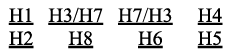Thus, H2 is the farthest from H4.
Hence, option 4.

IIFT Logical Reasoning MCQ Quiz - 2 - Question 19

Answer the following question based on the information given below.
Six classes, namely: Class A, Class B, Class C, Class D, Class E and Class F of a certain Management Institute were supposed to select exactly three specializations from Marketing, Finance, Human Resources, IT Management, Operations Management and Retail Management. All the students in any particular class selected the same set of specializations and further each specialization was selected by atleast one of the six classes. Also, 1. Students of Class B selected exactly one specialization which the students of Class E rejected. 2. There were exactly two specializations that were chosen by Class A as well as Class C. 3. There were exactly three specializations that were not chosen by Class C as well as Class F. 4. The students of Class F chose Marketing and Operations Management while the students of Class E did not choose IT Management.
The table below gives the details of the number of classes that opted for the specializations.In the above table: M: Marketing, F: Finance, H: Human Resources, I: IT Management, O: Operations Management.

Q.

Which number will fill the blank cell in the 4 x 4 matrix given below?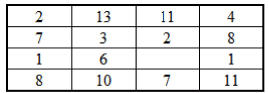Detailed Solution for IIFT Logical Reasoning MCQ Quiz - 2 - Question 19

The table has four rows and four columns.

In the first column, the sum of the numbers in the first two rows is the same as the sum of numbers in the last two rows i.e. 2 + 7= l + 8 = 9 This pattern is followed for all columns.

Similarly, in the first row, the sum of the numbers in the first two columns is the same as the sum of numbers in the last two columns i.e. 2+13 = 11+4 This pattern is also followed for all rows.

Hence, if the missing number is x, 11 + 2 =x + 7 and 1 + 6 = x + 1 x = 6 satisfies both the equations.

Hence, option 3.

IIFT Logical Reasoning MCQ Quiz - 2 - Question 20

In a music school, 38 students play the flute, 25 students play the violin and 30 students play the guitar. Also, 7 students play the flute and violin, 9 students play the violin and guitar and 12 students play the guitar and flute. The number of students who play exactly one instrument is 55. 15 students play only the violin. Every student plays at least one of these three instruments. How many students does this music school have?

Detailed Solution for IIFT Logical Reasoning MCQ Quiz - 2 - Question 20

The given data can be represented as shown below: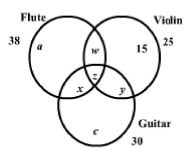a + 15 + c = 55 ...(i)

w + y + z + 15 = 25

∴  w + y + z = 10 ...(ii)

a + x + w + z = 38 ...(iii)

c + x + y + z = 30 ...(iv)

w + z = 7 ...(v)

y + z = 9...(vi)

x + z = 12 ...(vii)

Substituting (v) in (ii), y = 1 0 - 7 = 3

∴ z = 9 — 3 = 6

∴ w = 7 - 6 = 1 and x = 12 - 6 = 6

∴ c = 30 - (6 + 3 + 6) = 15

∴ a = 55 - ( 1 5 + 15) = 25

Thus, the entire Venn diagram can be filled as shown below: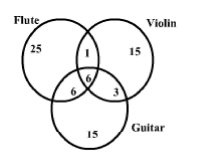∴ Total number of students = 25+ 15+ 15+ 1+ 6 + 3 + 6 = 71

Hence, option 2.

## IIFT Mock Test Series

1 docs|46 tests
 Use Code STAYHOME200 and get INR 200 additional OFF Use Coupon Code
Information about IIFT Logical Reasoning MCQ Quiz - 2 Page
In this test you can find the Exam questions for IIFT Logical Reasoning MCQ Quiz - 2 solved & explained in the simplest way possible. Besides giving Questions and answers for IIFT Logical Reasoning MCQ Quiz - 2, EduRev gives you an ample number of Online tests for practice

## IIFT Mock Test Series

1 docs|46 tests

### How to Prepare for CAT

Read our guide to prepare for CAT which is created by Toppers & the best Teachers